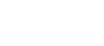# Integral of tan(x)

Maybe you know that the answer is ln(sec(x)), but do you know why? Here you’ll find the procedure with explanation!

First of all, let’s write the integral: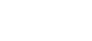We know that tan(x) can be written as sin(x)/cos(x), so: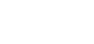Now, what do you think is the best method of integration for this integral? Notice that sin(x) is the derivative of cos(x) and that the derivative of cos(x) is -sin(x). If we let u=cos(x), we will have du=-sin(x) dx and so dx=-1/sin(x) du. This way the sin(x) at the nominator cancels out with the sin(x) at the denominator of dx. Yes, I am talking about u-substitution!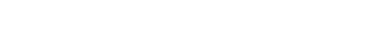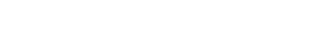At this point we can undo u-sub (remember that u=cos(x):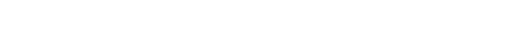I used the absolute value for the argument of the logarithm because, in order to get real numbers, it can’t be negative. Also, -ln(cos(x)) becomes ln(sec(x)) because the number multiplied by ln, in this case -1, is the exponent of the argument. So, if you have ln(x²), you can write it as 2ln(x) and vice versa. (cos(x))^-1 is equal to 1/cos(x) which is sec(x).

Overall, we have: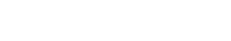And that’s it!

Now, give this a try!